# NCERT Solutions For Class 10 Maths Chapter 10 Exercise 10.2

## Chapter 10 Ex.10.2 Question 1

From a point $$Q,$$ the length of the tangent to a circle is $$\text{24 cm}$$ and the distance of $$Q$$ from the center is $$\text{25 cm.}$$

The radius of the circle is

(A)    $$\text{7 cm}$$

(B)     $$\text{12 cm}$$

(C)     $$\text{15 cm}$$

(D)     $$\text{24.5 cm}$$

### Solution

What is Known?

(i) Length of tangent from a point $$Q,$$ i.e. $$PQ$$ is $$\text{24 cm}$$

(ii) Distance of $$Q$$ from center, i.e. $$OQ$$ is $$\text{25 cm.}$$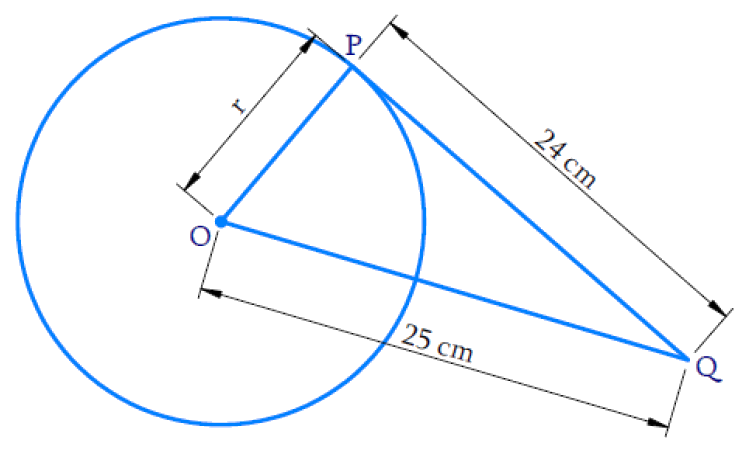What is Unknown?

Radius of the circle

Reasoning:

Tangent at any point of a circle is perpendicular to the radius through the point of contact.

Steps:

$$\therefore \; \Delta \,{OPQ}$$ is a right-angled triangle

By Pythagoras theorem,

\begin{align} {O Q} ^ { 2 } &= {O P} ^ { 2 } + {P Q} ^ { 2 } \\ 25 ^ { 2 } &= r ^ { 2 } + 24 ^ { 2 } \\ r ^ { 2 } &= 25 ^ { 2 } - 24 ^ { 2 } \\ & = 625 - 576 \\ r ^ { 2 } &= 49 \\ r& = \pm 7 \end{align}

Radius cannot be a negative value,

$$\therefore\; r = + 7 \; \rm{cm}$$

Hence the correct  Option is A

## Chapter 10 Ex.10.2 Question 2

In the given figure, if $$TP$$ and $$TQ$$ are the two tangents to a circle with centre $$O$$ so that $$\angle {P O Q} = 110 ^ { \circ }$$, then $$\angle {P T Q}$$ is equal to

(A)$$60^\circ$$

(B)$$70^\circ$$

(C)$$80^\circ$$

(D)$$90^\circ$$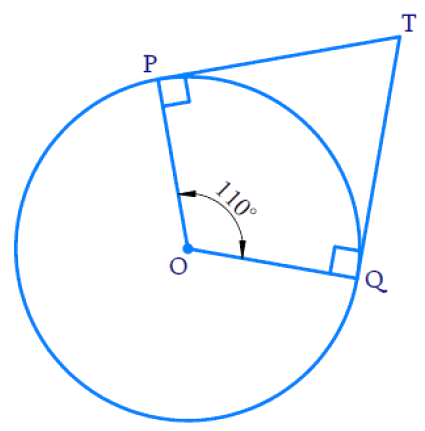### Solution

What is Known?

(i) $$TP$$ and $$TQ$$ are tangents to a circle with Centre $$O$$

(ii) $$\angle {P O Q}=110^{\circ}$$

What is Unknown?

$$\angle {PTQ}$$

Reasoning:

• Tangent at any point of a circle is  perpendicular to the radius through the point of contact.
• In the above figure $$OPTQ$$ is a quadrilateral and $$\angle {P}$$ and $$\angle {Q}$$  are $$90^{\circ}$$
• Sum of the angles of a quadrilateral is $$360^{\circ}$$

Steps:

$$\therefore \;$$In $$OPTQ,$$

\begin{align} \left[ \begin{array} & \angle Q+\angle P+ \\ \angle POQ+\angle PTQ \\ \end{array} \right]&\!=\!{{360}^{{}^\circ }} \\ \left[ \begin{array} & {{90}^{{}^\circ }}+{{90}^{{}^\circ }}+ \\ {{110}^{{}^\circ }}+\angle PTQ \\ \end{array} \right]&\!=\!{{360}^{{}^\circ }} \\ {{290}^{{}^\circ }}+\angle PTQ&\!=\!{{360}^{{}^\circ }} \\ \angle PTQ&\!=\!{{360}^{{}^\circ }}-{{290}^{{}^\circ }} \\ \angle PTQ&\!=\!{{70}^{{}^\circ }} \\ \end{align}

Hence the correct Option is B

## Chapter 10 Ex.10.2 Question 3

If tangents $$PA$$ and $$PB$$ from a point $$P$$ to a circle with center $$O$$ are inclined to each other at angle of $$80^\circ,$$ then $$\angle {POA}$$ is equal to

(A)$$50^\circ$$

(B)$$60^\circ$$

(C)$$70^\circ$$

(D)$$80^\circ$$

### Solution

What is Known?

$$PA$$ and $$PB$$ are the tangents from $$P$$ to a circle with center $$O.$$ Tangents are inclined to each other at angle of $$80^\circ$$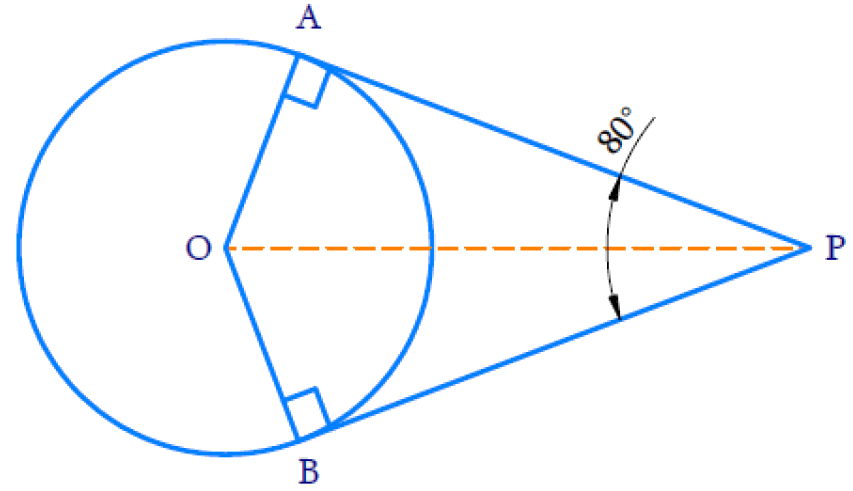What is Unknown?

$$\angle {POA}$$

Reasoning:

• The lengths of tangents drawn from an external point to a circle are equal
• Tangent at any point of a circle is perpendicular to theradius through the point of contact.

Steps:

In $$\triangle {OAP}$$ and in $$\triangle {OBP}$$

$$OA = OB$$ (radii of the circle are always equal)

$$AP = BP$$ (length of the tangents)

$$OP = OP$$ (common)

Therefore, by $$SSS$$ congruency $$\Delta {OAP} \cong \Delta {O B P}$$

SSS congruence rule: If three sides of one triangle are equal to the three sides of another triangle, then the two triangles are congruent.

If two triangles are congruent then their corrresponding pars are equal.

Hence,

$$\angle POA = \angle POB \\\angle OPA = \angle OPB$$

Therefore, $$OP$$ is the angle bisector of $$\angle {APB}$$ and $$\angle {AOB}$$

\begin{align} \therefore \quad \angle {O P A}&= \angle O P B\\& = \frac { 1 } { 2 } ( \angle A P B ) \\ & = \frac { 1 } { 2 } ( 80 ) \\ & = 40 ^ { \circ } \end{align}

By angle sum property of a triangle, In $$\Delta {OAP}$$

$\angle {A} + \angle {POA} + \angle {OPA} = 180 ^ { \circ }$

From the figure,

$${OA} \, \bot \, {AP}$$ (Theorem 10.1: The tangent at any point of a circle is perpendicular to the radius through the point of contact.)

\begin{align} \therefore \quad \angle {A} &= 90 ^ { \circ } \\ 90 ^ { \circ } + \angle { POA } + 40 ^ { \circ } &= 180 ^ { \circ } \\ 130 ^ { \circ } + \angle { POA }& = 180 ^ { \circ } \\ \angle { POA } & = 180 ^ { \circ } - 130 ^ { \circ } \\ \angle { POA } & = 50 ^ { \circ } \end{align}

The correct Option is A

## Chapter 10 Ex.10.2 Question 4

Prove that the tangents drawn at the ends of a diameter of a circle are parallel.

### Solution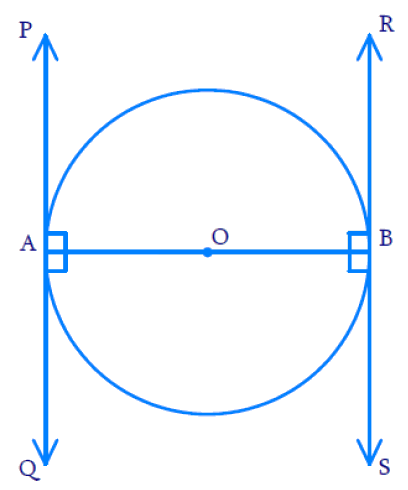What is known?

• Let $$AB$$ be a diameter of the circle.
• Two tangents $$PQ$$ and $$RS$$ are drawn at points $$A$$ and $$B$$ respectively.

To Prove:

Tangents drawn at the ends of a diameter of a circle are parallel.

Reasoning :

• A tangent to a circle is a line that intersects the circle at only one point.
• Theorem 10.1 : The tangent at any point of a circle is perpendicular to the radius through the point of contact.

Steps:

We know that, according to Theorem 10.1, Radius is perpendicular to the tangent at the point of contact

Thus, $${OA}\, \bot \, {PQ}$$ and $${OB}\, \bot\, {Z}$$

Since the Tangents are Perpendicular to the Radius,

\begin{aligned} \angle {P A O} & = 90 ^ { \circ } \\ \angle {R B O} & = 90 ^ { \circ } \\ \angle {O A Q} & = 90 ^ { \circ } \\ \angle {O B S} & = 90 ^ { \circ } \end{aligned}

Here $$\angle {OAQ}$$ & $$\angle {OBR}$$ and $$\angle {PAO}$$ & $$\angle {OBS}$$ are two pairs of alternate interior angles and they are equal.

If the Alternate interior angles are equal then lines $$PQ$$ and $$RS$$ should be parallel.

We know that $$PQ$$ & $$RS$$ are the tangents drawn to the circle at the ends of the diameter $$A B.$$

Hence, it is proved that Tangents drawn at the ends of a diameter of a circle are parallel.

## Chapter 10 Ex.10.2 Question 5

Prove that the perpendicular at the point of contact to the tangent to a circle passes through the center.

### Solution

To prove:

Perpendicular at the point of contact to the tangent to a circle passes through the centre.

Reasoning and Steps: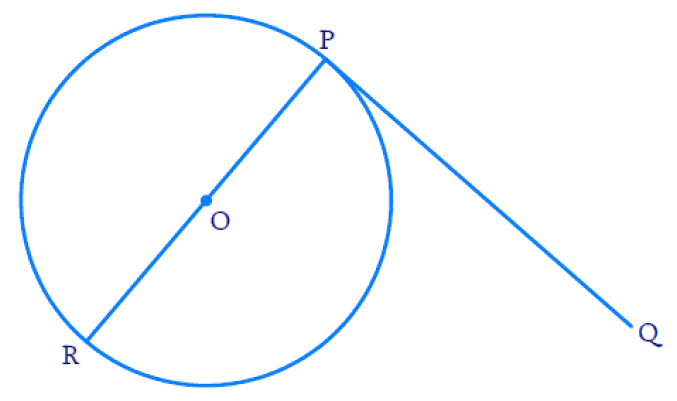By theorem, tangent at any point of a circle is perpendicular to the radius through the point of contact.

Radius will always pass through the center of the circle.

Hence proved that perpendicular $$PR$$ of tangent $$PQ$$ passes through center $$O.$$

## Chapter 10 Ex.10.2 Question 6

The length of a tangent from a point $$A$$ at distance $$\text{5 cm}$$ from the center of the circle is $$\text{4 cm.}$$ Find the radius of the circle.

### Solution

What is Known?

Length of tangent from point $$A = \text{4 cm.}$$

Distance between the centre of the circle and point $$A$$ is $$\text{5 cm.}$$

What is Unknown?

Radius of the circle

Reasoning: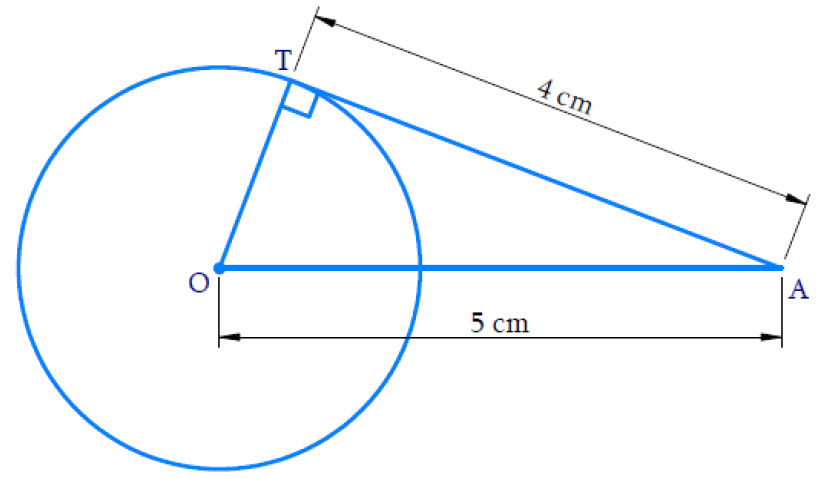Tangent at any point of a circle is perpendicular to the radius through the point of contact.

Therefore, $$\angle {OTA} = {90^ \circ }$$ and $$\Delta {OTA}$$ is right angled triangle.

Steps:

By Pythagoras theorem

\begin{align} {O A} ^ { 2 } &= {O T} ^ { 2 } + {A T} ^ { 2 } & \\ 5 ^ { 2 } & = {O T} ^ { 2 } + 4 ^ { 2 } \\ {O T} ^ { 2 } & = 25 - 16 \\ {O T} ^ { 2 } & = 9 \\ {O T} & = \pm 3 \end{align}

Radius $$OT$$ cannot be negative. Hence, the radius is $$\text{3 cm.}$$

## Chapter 10 Ex.10.2 Question 7

Two concentric circles are of radii $$\text{5 cm}$$ and $$\text{3 cm.}$$ Find the length of the chord of the larger circle which touches the smaller circle.

### Solution

What is Known?

Two concentric circles are of radii $$\text{5 cm}$$ and $$\text{3 cm.}$$

What is Unknown?

The length of the chord of the larger circle which touches the smaller circle.

Reasoning:

Chord of the larger circle is a tangent to the smaller circle.

Steps: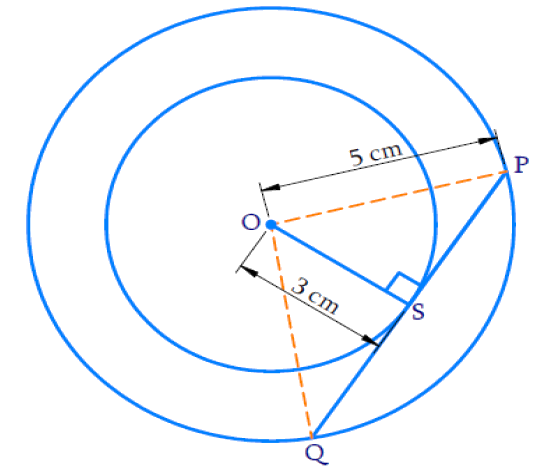$$PQ$$ is chord of a larger circle and tangent of a smaller circle. Tangent is perpendicular to the radius at the point of contact $$S.$$

$$\therefore \;\angle {OSP} = {90^ \circ }$$

In $$\Delta {OSP}$$ (Right angled triangle)

By the Pythagoras Theorem,

\begin{align} {O P} ^ { 2 } & = {O S} ^ { 2 } + {S P} ^ { 2 } \\ 5 ^ { 2 } & = 3 ^ { 2 } + {S P} ^ { 2 } \\ {S P} ^ { 2 } & = 25 - 9 \\ {S P} ^ { 2 } & = 16 \\ {S P} & = \pm 4 \end{align}

$$SP$$ is length of tangent and cannot be negative

$$\therefore \;SP = \rm{4\, cm}$$

$$QS = SP$$ (Perpendicular from center bisects the chord considering the larger circles)

Therefore, $$QS = SP = \text{4 cm}$$

Length of the chord

\begin{align} {PQ} & = \text{QS + SP} \\ & = 4 + 4 \\ { PQ} & = 8 \; \rm{ cm }\end{align}

Therefore, the length of the chord of the larger circle is $$\text{8 cm.}$$

## Chapter 10 Ex.10.2 Question 8

A quadrilateral $$ABCD$$ is drawn to circumscribe a circle (see given Figure). Prove that: $$AB + CD = AD + BC$$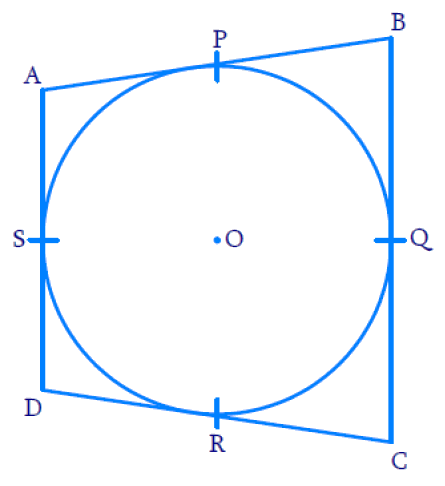### Solution

To prove:

$$AB + CD = AD + BC$$

Reasoning:

• A tangent to a circle is a line that intersects the circle at only one point.
• Theorem 10.1: The tangent at any point of a circle is perpendicular to the radius through the point of contact.
• Theorem 10.2: The lengths of tangents drawn from an external point to a circle are equal.

Steps: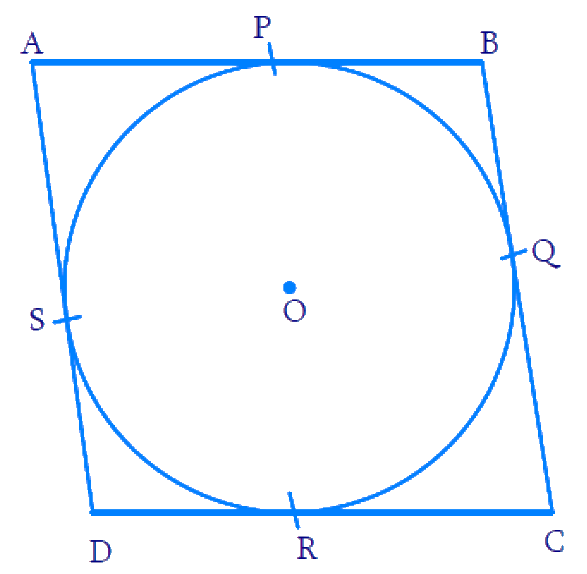We know that the length of tangents drawn from an external point of the circle are equal according to Theorem $$10.2.$$

Therefore,

$$BP = BQ$$ (Tangents from point $$B$$) $$\dots\dots\rm{(i)}$$

$$CR = CQ$$ (Tangents from point $$C$$) $$\dots \dots\rm{(ii)}$$

$$DR = DS$$  (Tangents from point $$D$$) $$\dots \dots\rm{(iii)}$$

$$AP = AS$$ (Tangents from point $$A$$$$\dots \dots\rm{(iv)}$$

Adding all the four equations, $$\text{( i) +( ii) + (iii )+ (iv)}$$ we get,

\begin{align}BP + CR + DR + AP \\= BQ + CQ + DS + AS\end{align}

On re-grouping them,

\begin{align}( A P + B P ) + ( C R + D R ) = \\( A S + D S ) + ( B Q + C Q )\, .... \,( \rm{v} )\end{align}

From the figure, we can see that:

\begin{align} {AP + BP} &= {AB} \\ {CR + DR} &= {CD} \\{AS + DS} &= {A D}\\{BQ + CQ} &= {B C}\end{align}

On substituting the above values in $$\rm{(v),}$$ we get

$$AB + CD = AD + BC$$

Hence Proved.

## Chapter 10 Ex.10.2 Question 9

In Figure, $$XY$$ and $$X'Y'$$ are two parallel tangents to a circle with center $$O$$ and another tangent $$AB$$ with point of contact $$C$$ intersecting $$XY$$ at $$A$$ and $$X'Y'$$ at $$B.$$

Prove that $$\angle {AOB} = {90^ \circ }$$.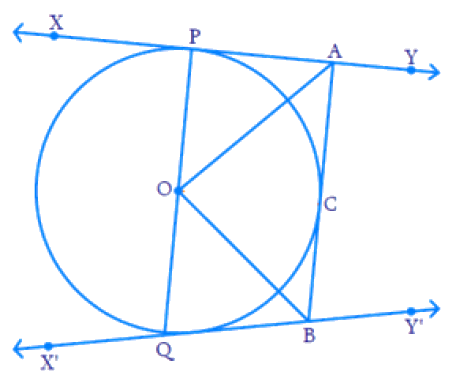### Solution

What is known?

•  '$$O$$' is the centre of the circle.
• $$XY$$ and $$X'Y'$$' are the two parallel tangents to the circle.
• $$AB$$ is another tangent with point of contact $$C$$, intersecting $$XY$$ at $$A$$ and $$X'Y'$$ at $$B.$$

To prove:

$$\angle {AOB} = {90^ \circ }$$

Reasoning :

Join point $$O$$ to $$C.$$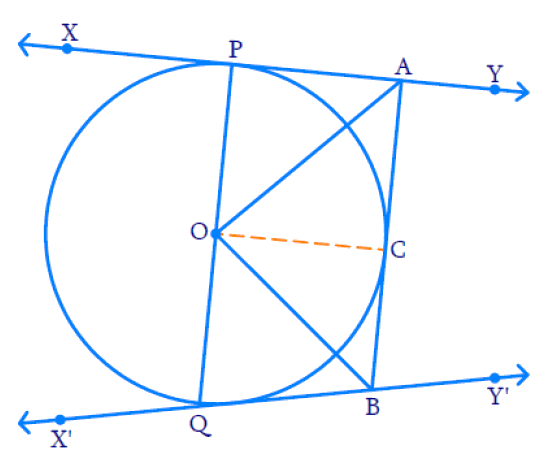In $$\Delta OPA$$ and $$\Delta OCA$$

$$OP = OC$$ (Radii of the circle are equal)

$$AP = AC$$ (The lengths of tangents drawn from an external point $$A$$ to a circle are equal.)

$$AO = AO$$  (Common)

By $$SSS$$ congruency, $$\Delta {OPA} \cong \Delta {OCA}$$

SSS Congruence Rule: If three sides of one triangle are equal to the three sides of another triangle, then the two triangles are congruent.

Steps:

Therefore, $$\angle {OPA} = \angle {OCA}$$

Similarly, $$\Delta {OCB} \cong \Delta {OBQ}$$

Therefore, $$\angle {COB} = \angle {BOQ}$$

$$PQ$$ is a diameter, hence a straight line and $$\angle {POQ} = {180^ \circ}$$

But \begin{align} \angle {P O Q} =\begin{bmatrix} \angle {P O A} + \angle {A O C}+ \\ \angle {C O B} + \angle {B O Q}\end{bmatrix}\end{align}

Therefore,
\begin{align} & \left[ \begin{array} \angle POA+\angle AOC+ \\ \angle COB+\angle BOQ \\ \end{array} \right]={{180}^{{}^\circ }} \\ & 2\angle AOC+ 2\angle COB ={{180}^{{}^\circ }}\end{align}

Therefore,
\begin{align}& \left[ \begin{array}& \angle POA=\angle AOC \;\text{and} \\\angle COB=\angle BOQ \\ \end{array} \right] \end{align}

Therefore,
$\angle AOC+\angle COB={{90}^{{}^\circ }}$

From the figure, $$\angle {A O C} + \angle {C O B} = \angle {A O B}$$

$$\therefore \quad \angle {A O B} = 90 ^ { \circ }$$

Hence Proved

## Chapter 10 Ex.10.2 Question 10

Prove that the angle between the two tangents drawn from an external point to a circle is supplementary to the angle subtended by the line-segment joining the points of contact at the center.

### Solution

What is Known?

• Let us consider a circle centered at point $${O.}$$
• Let $${P}$$ be an external point from which two tangents $${PA}$$ and $${PB}$$ are drawn to the circle which are touching the circle at point $${A}$$ and $${B}$$ respectively
• $${AB}$$ is the line segment, joining point of contacts $${A}$$ and $${B}$$ together such that it subtends $$\angle {AOB}$$ at center $${O}$$ of the circle.

To prove:

The angle between the two tangents drawn from an external point to a circle is supplementary to the angle subtended by the line segment joining the point of contact at the center.

i.e. $$\angle {APB}$$ is supplementary to $$\angle {AOB}$$

Reasoning: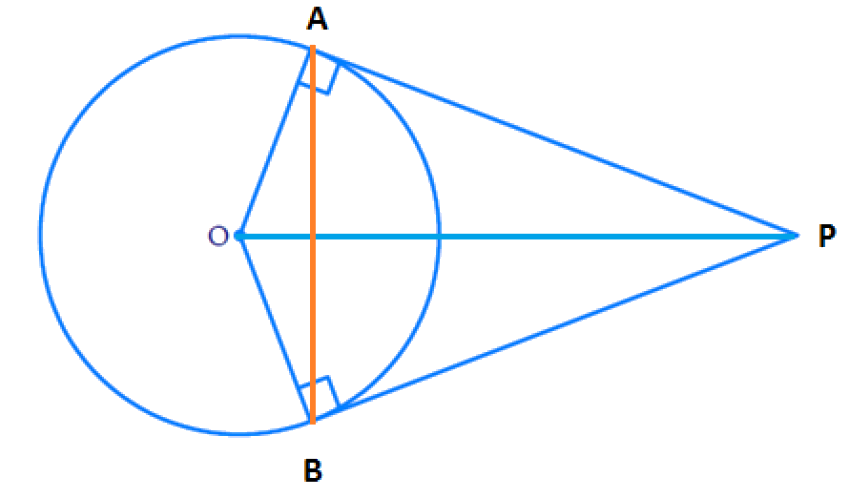According to Theorem 10.1: The tangent at any point of a circle is perpendicular to the radius through the point of contact.

\begin{align}\therefore \angle {OAP} = \angle {OBP} = 90^{ \circ } \ldots \end{align}  Equation (i)

Steps :

In a quadrilateral, sum of 4 angles is $${360^ \circ }$$

$$\therefore$$ In $${OAPB}$$,

\begin{align}\begin{bmatrix}\angle {OAP} + \angle {APB} + \\\angle {PBO} + \angle {BOA} \end{bmatrix}= 360 ^ { \circ }\end{align}

Using Equation (i), we can write the above equation as

\begin{align} \left[ \begin{array} & {{90}^{{}^\circ }}+\angle APB+ \\ {{90}^{{}^\circ }}+\angle BOA \\ \end{array} \right]&\!\!=\!{{360}^{^\circ }} \\ \angle APB+\angle BOA&\!\!=\!{{360}^{{}^\circ }}\!\!-\!\!{{180}^{{}^\circ }} \\\therefore \angle APB+\angle BOA&\!\!=\!\!{{180}^{{}^\circ }} \\ \end{align}

Where, $$\angle {APB}=$$ Angle between the two tangents $${PA}$$ and $${PB}$$ from external point $${P}$$.

$$\angle {BOA}=$$ Angle subtended by the line segment joining the point of contact at the center.

Hence Proved.

## Chapter 10 Ex.10.2 Question 11

Prove that the parallelogram circumscribing a circle is a rhombus.

### Solution

To prove:

The parallelogram circumscribing a circle is a rhombus

Reasoning:$${ABCD}$$ is a parallelogram. Therefore, opposite sides are equal.

\begin{align}\therefore {AB} & = {CD} \\ {BC} & = {AD} \end{align}

According to Theorem 10.2 : The lengths of tangents drawn from an external point to a circle are equal.

Steps :

Therefore,

$$BP = BQ$$ (Tangents from point $$B$$)…… ($$1$$)

$$CR = CQ$$ (Tangents from point $$C$$)…… ($$2$$)

$$DR = DS$$ (Tangents from point $$D$$)…… ($$3$$)

$$AP = AS$$ (Tangents from point $$A$$)……. ($$4$$)

Adding $$(1) + (2) + (3) + (4)$$

\begin{align} & BP+CR+DR+AP \\ & =BQ+CQ+DS+AS \\ \end{align}
On re-grouping,

\begin{align} \left[ \begin{array} & BP+AP+ \\ CR+DR \\ \end{array} \right]&=\left[ \begin{array} & BQ+CQ+ \\ DS+AS \\ \end{array} \right] \\ AB+CD&=BC+AD \\ \end{align}

Substitute $${CD = AB}$$ and $${AD = BC}$$ since $${ABCD}$$ is a parallelogram, then

\begin{align}{ A B + A B} & = {B C + B C} \\ {2 A B} & ={ 2 B C} \\ {A B} & = {BC} \end{align}

$$\therefore {AB=BC=CD=DA}$$

This implies that all the four sides are equal.

Therefore, the parallelogram circumscribing a circle is a rhombus.

## Chapter 10 Ex.10.2 Question 12

A triangle $$ABC$$ is drawn to circumscribe a circle of radius $$4\, \rm{cm}$$ such that the segments $$BD$$ and $$DC$$ into which $$BC$$ is divided by the point of contact $$D$$ are of lengths $$8 \,\rm{cm}$$ and $$6\, \rm{cm}$$ respectively (see Figure). Find the sides $$AB$$ and $$AC$$.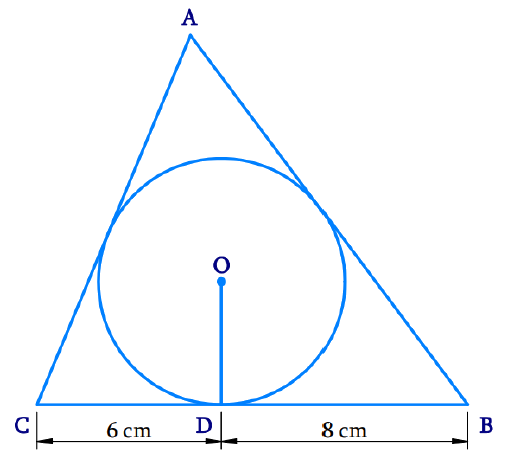### Solution

What is the known?

(i) $$\Delta {ABC}$$ is drawn to circumscribe a circle of radius $$4\, \rm{cm.}$$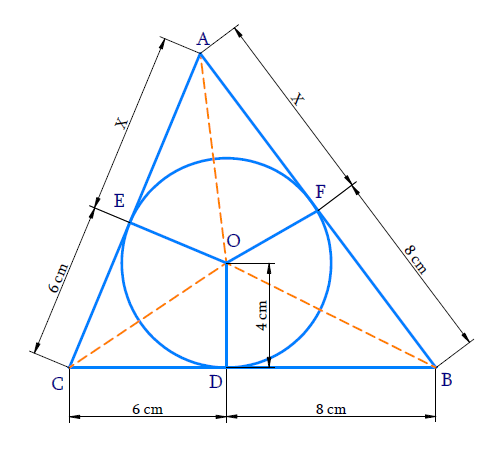\begin{align}{BD}&=8\;\rm{cm}\\{CD}&=6\;\rm{cm}\end{align}

What is Unknown?

Sides $${AB}$$ and $${AC}$$

Reasoning:

Finding the area of $$\Delta {ABC}$$ in two ways and equating them will result in unknown length. Hence other sides can be calculated.

Let,

$${AE = AF = x}$$ (The lengths of tangents drawn from an external point to a circle are equal.)

$${CD = CE = 6 \rm cm}$$ (Tangents from point $$C$$)

$${BD = BF = 8 \rm{cm}}$$ (Tangents from point $$B$$)

Area of the triangle

\begin{align}= \sqrt {{ s ( s - a ) ( s - b ) ( s - c )} } \end{align}

where \begin{align} s = \frac { 1 } { 2 } ( a + b + c )\end{align}

$$a,\; b$$ and $$c$$ are sides of a triangle

\begin{align} a &= AB= x + 8 \\ b &= BC = 8 + 6 \\ & = 14 \\c& = CA = 6 + x \end{align}

\begin{align} s & = \frac { 1 } { 2 } ( x + 8 + 14 + 6 + x ) \\ s & = \frac { 1 } { 2 } ( 2 x + 28 ) \\ s & = x + 14 \end{align}

Area of $$\Delta {ABC}$$

\begin{align} & = \sqrt { (x + 14) ( x + 14 - x - 8 ) ( x + 14 - 14 ) ( x + 14 - x + 6 ) } \\ & = \sqrt { ( x + 14 ) ( 6 ) ( x ) ( 8 ) } \\ & = \sqrt { 48 x ( x + 14 ) } \\ & = \sqrt { 48 \left( x ^ { 2 } + 14 x \right) }\;\text {sq units}\dots\text{Equation (1)} \end{align}

Area of $$\Delta ABC=$$ Area of $$\Delta {AOC}\;+\;$$Area of $$\Delta {AOB}\;+\;$$ Area of $$\Delta {BOC}$$

\begin{align} & = \frac { 1 } { 2 } ( x + 6 ) 4 + \frac { 1 } { 2 } ( x + 8 ) 4 + \frac { 1 } { 2 } ( 14 ) 4 \\& \left(\therefore \operatorname{area~of} \Delta=\frac{1}{2} \times b \times h\right)\\ & = 2 x + 12 + 2 x + 16 + 28 \\ & = 4 x + 56 \\ & = 4 ( x + 14 ) \text{ sq units}\dots\text{ (Equation 2)} \end{align}

Equating (1) and (2) $\sqrt { 48 \left( x ^ { 2 } + 14 x \right) } = 4 ( x + 14 )$

Squaring both sides

\begin{align} 48 ( x ^ 2 + 14 x ) & = 4 ^ { 2 } ( x + 14 ) ^ { 2 } \\ 48 ( x ^ { 2 } + 14 x ) & = 16 ( x ^ { 2 } + 28 x + 196 ) \\ [\text{Using }(a+b)^{2}&={a^{2}+2 a b+b^{2}}] \\ \frac { 48 } { 16 } ( x ^ { 2 } + 14 x ) & = x ^ { 2 } + 28 x + 196\\ 3 x^{2}+42 x &=x^{2}+28 x+196 \\ \begin{bmatrix}3 x ^ { 2 } - x ^ { 2 } +42 \\ x -28 x - 196 \end{bmatrix} & = 0 \\ 2 x ^ { 2 } + 14 x - 196 & = 0\\ \text{(divide this } & \text{equation by 2)} \\ x ^ { 2 } + 7 x - 98 & = 0 \end{align}

Solving by factorization method

\begin{align} x ^ { 2 } + 14 x - 7 x - 98 & = 0 \\ x ( x + 14 ) - 7 ( x + 14 ) & = 0 \\ ( x + 14 ) ( x - 7 ) & = 0 \end{align}

\begin{align} &{ x + 14 = 0 } \qquad\text{and} \qquad x - 7 = 0 \\ &{ x = - 14 } \qquad \qquad \qquad \;\; { x = 7 } \end{align}

Since $$x$$ represents length, it cannot be negative.

$$\therefore x = 7$$

\begin{align}AB = a = x + 8 = 7 + 8 = 15 \,\rm{cm} \\ AC = c = 6 + x = 6 + 7 = 13\,\rm{cm} \end{align}

Hence the sides

$$AB = 15\, \rm{cm}\\AC = 13 \,\rm{cm}$$

## Chapter 10 Ex.10.2 Question 13

Prove that opposite sides of a quadrilateral circumscribing a circle subtend supplementary angles at the center of the circle.

### Solution

To prove:

Opposite sides of a quadrilateral circumscribing a circle subtend supplementary angles at the center of the circle.

Steps and Reasoning: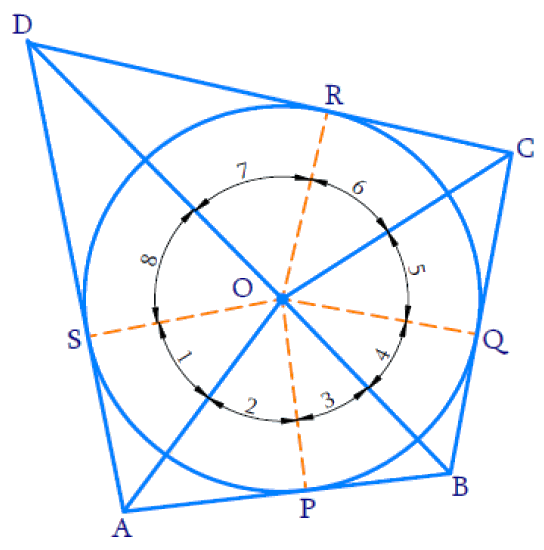We know that, tangents drawn from an external point to a circle subtend equal angles at the center.

In the above figure, $${P,\;Q,\;R,\;S}$$ are point of contacts

$${AS = AP}$$ (The lengths of tangents drawn from an external point $$A$$ to a circle are equal.)

\begin{align} \angle {SOA} = \angle {POA} = \angle 1 = \angle 2 \end{align}

Tangents drawn from an external point to a circle subtend equal angles at the centre.

Similarly,

\begin{align} \angle 3 = \angle 4 , \angle 5 = \angle 6 , \angle 7 = \angle 8 \end{align}

Since complete angle is $${360^ \circ }$$,

\begin{align}\left[ \begin{array} & \angle 1+\angle 2+\angle 3+\angle 4+ \\ \angle 5+\angle 6+\angle 7+\angle 8 \\ \end{array} \right]&={{360}^{^\circ }} \\2(\angle 1+\angle 8+\angle 4+\angle 5)&={{360}^{^\circ }} \\ \,\angle 1+\angle 8+\angle 4+\angle 5&={{180}^{{}^\circ }} \\ \end{align}

[or]

\begin{align} 2(\angle 2+\angle 3+\angle 6+\angle 7) &=360^{\circ} \\ \angle 2+\angle 3+\angle 6+\angle 7 &=180^{\circ} \\ \angle A O B+\angle C O D &=180^{\circ} \end{align}

From above figure \begin{align}\angle A O D+\angle B O C =180^{\circ}\end{align}

$$\angle {AOD}$$ and $$\angle {BOC}$$ are angles subtended by opposite sides of quadrilateral circumscribing a circle and sum of them is $${180^ \circ }$$

Hence proved.

Download SOLVED Practice Questions of NCERT Solutions For Class 10 Maths Chapter 10 Exercise 10.2 for FREE
Ncert Class 10 Exercise 10.2
Ncert Solutions For Class 10 Maths Chapter 10 Exercise 10.2
Instant doubt clearing with Cuemath Advanced Math Program